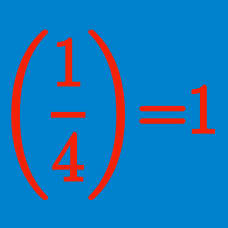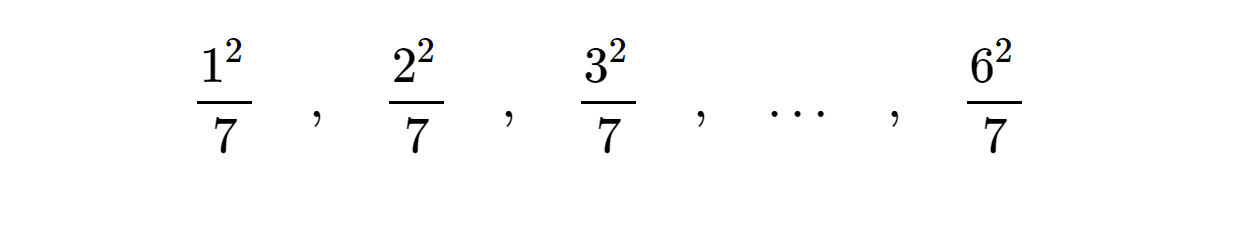Number Theory

#### Concept QuizzesIs 5 a quadratic residue modulo 7?

Which of these is the set of non-quadratic residues modulo $11$?

Which of these is the set of quadratic residues modulo $15$?

Find the sum of all positive integers $x<22$ such that $x^2 \equiv 17 \pmod {22}$.

Is $15$ a quadratic residue modulo $17$?

×